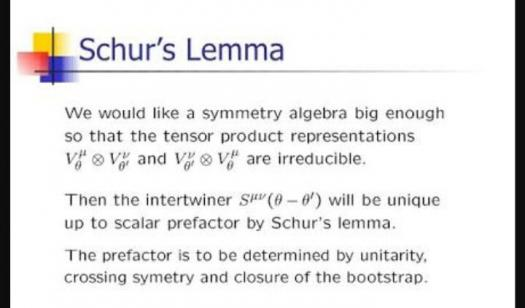# What Do You Know About Schur's Lemma?

10 Questions | Total Attempts: 126SettingsIn the representation theory branch of mathematics, Schur's lemma is a popular theorem used for algebras and groups. Despite the fact that the theorem is outright basic, it has been very useful since it was created in the 20th century. For groups and algebras, the theorem uses different approaches. To find out more about Schur's lemma, sit back and enjoy the following questions.

• 1.
How do we determine if a ring is strongly indecomposable?
• A.

If its endomorphism ring is a local ring

• B.

If its exomorphism ring is a local ring

• C.

If the external ring is a designer ring

• D.

If it has a well-designed and structured ring

• 2.
What is the property of a module of a division ring?
• A.

Decomposable

• B.

Indecomposable

• C.

Compressed state

• D.

Precipitation

• 3.
Which one of the following representation theories do we use the theorem for?
• A.

Simultaneous equations

• B.

Jeff theory

• C.

Linear equations

• D.

Algebra

• 4.
What does the sign φ represent?
• A.

Linear representation

• B.

Geometrical representation

• C.

Non-linear representation

• D.

Planar representation

• 5.
Who discovered the theorem?
• A.

Isaac Shcur

• B.

Issai Schur

• C.

John Schur

• D.

Michael Schur

• 6.
In the theorem, what is the property of V and W?
• A.

Isothermic

• B.

Isomorphic

• C.

Amorphotic

• D.

Geometric

• 7.
In the theorem, what do V and W represent?
• A.

Scalar spaces

• B.

Vector spaces

• C.

Geometric representation

• D.

Concentric spaces

• 8.
What happens when V=W?
• A.

Trivial G-linear maps become the identity

• B.

Nontrivial G-linear maps become the identity

• C.

The linear map changes its position

• D.

More linear maps would be formed

• 9.
If φ is invertible, what would φ be equal to?
• A.

-1

• B.

0

• C.

1

• D.

0.1

• 10.
How do we determine that Φ is not bijective?
• A.

Ran(Φ) is larger than N

• B.

Ran(Φ) does not exist

• C.

Ran(Φ) is equal to N

• D.

Ran(Φ) is smaller than N

Related TopicsBack to top